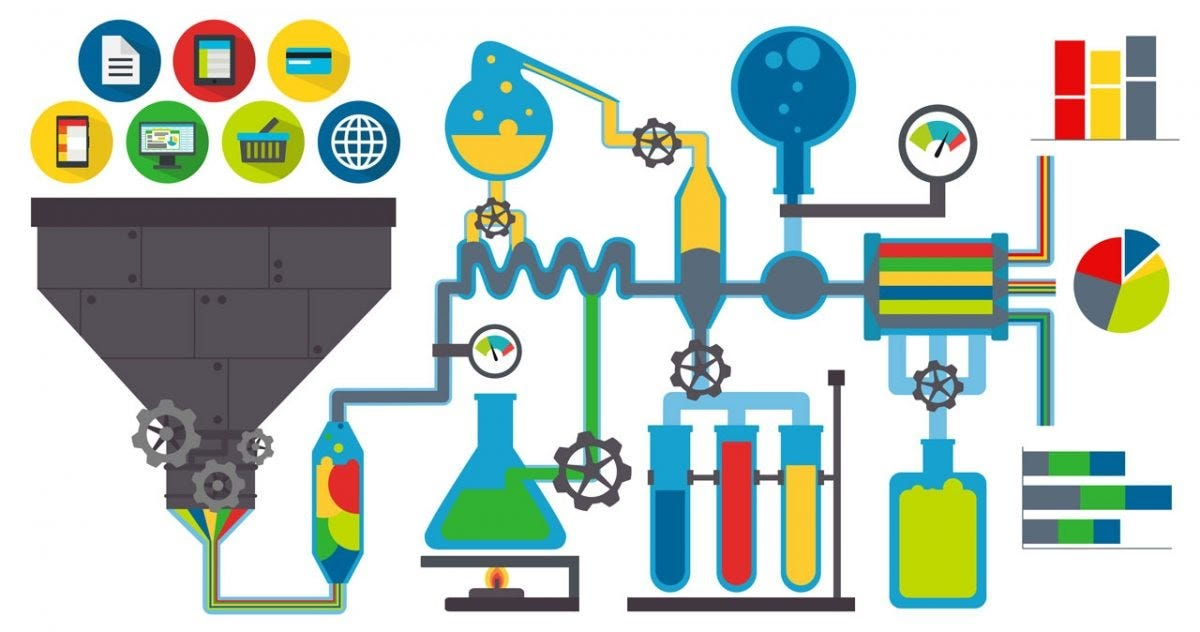# Six steps to hone your Data: Data Preprocessing, Part 5What is the train set?

The sample of data used to fit the model is called a train set.

We will train our Machine Learning models on the train set, which means that our model will try to find correlations present in this train set and evaluate it later.

Our model will capture insights and learn from this data.

What is a test set?

The sample of data used to provide an unbiased evaluation of a final model fit on the training dataset is called a test set.

Whatever our model has learned from the train set is tested on the test set. We do not want our model to overfit, but we want it to learn.

The test set is generally well-curated. It contains carefully sampled data that spans the various classes that the model would face when used in the real world.

Thus whatever insights our model has gained through train sets are tested in the test set.

Note — A part of the dataset also needs to be split into a Validation Set.

The validation set is a training set in which hyperparameters of the dataset are tuned.

Since we have no clue of hyperparameters yet, we will come to it shortly.

Now that we are clear about the concept of the train test and test set, we need to think about in what parts do we split our dataset?

What is the dataset split ratio?

The split ratio is dependant on two things:

• The number of data samples you have.
• The actual model you want to train.

Some models need a substantial amount of data for training, and thus, in that case, we need a training set bigger than a test set. Usually, as you build more and more models, you begin to get a grasp on the ratio of splits required.

But since we haven’t built any model yet, we will follow the general splitting technique.

Usually, the dataset is split into a 70:30 ratio or 80:20 ratio.

Thus, you either take 70% or 80% of the data as a train set while leaving out the rest 30% or 20% as a test set.

How to split a dataset?

To carry out this split, we will import test_train_split from the model_selection library of sci-kit learn.

I will be demonstrating Dataset split using the following dataset :

Let us consider this dataset, import all the necessary libraries, import our dataset, and separate it into features and targets, handle missing data, and encode categorical data.

Now, we will split our dataset into an 80% train set and a 20% test set.

We need four sets to build our training set and testing set, as follows:

1. X_train (training part of the matrix of features)
2. X_test (test part of the matrix of features)
3. y_train (training part of the target variables associated with the X train sets)
4. y_test (test part of the target variables associated with the X test sets)

We will assign to them the test_train_split, which takes the following parameters:

• Arrays (X,y)
• test_size (if we give it the value 0.2, meaning 20%, it will split the dataset into a 1–5th part.
• Since an ideal choice is to allocate 20% of the dataset to the test set, the test_set is set to 0.2).

The code snippet below shows how to do this.

Congratulations! You can now split a dataset into a train and test set. We are only one step from finishing off our data processing series.

The efforts you put in honing your data reflect through your model. Keep practicing peep!